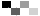# LettermazeR N B Z S U V M F D A C U P R M F U P P R M Y S B D U Y Y F L E S Z W M P G R Y L N I U R Y D J T F T G T P O X N Q K I H U O C T Q Y X P M W A Y A G J W L K D N S R U K B M U F W R E F D T Y E A O

LENGTHWAYS REFRACTORY

Memorize the 2 words – then spell them by moving your mouse through the maze.

"IQ" Score: 0